## Lerch Transcendent

A generalization of the Hurwitz Zeta Function and Polylogarithm function. Many sums of reciprocal Powers can be expressed in terms of it. It is defined by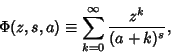(1)

where any term with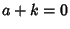is excluded.

The Lerch transcendent can be used to express the Dirichlet Beta Function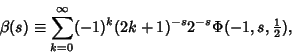(2)

the integral of the Fermi-Dirac Distribution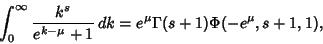(3)

where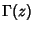is the Gamma Function, and to evaluate the Dirichlet L-Series.

See also Dirichlet Beta Function, Dirichlet L-Series, Fermi-Dirac Distribution, Hurwitz Zeta Function, Polylogarithm